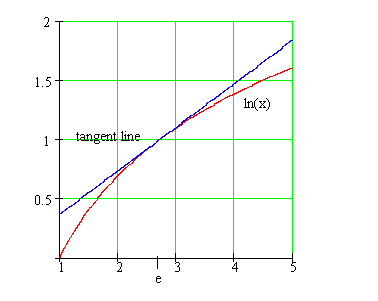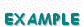Calculus on Demand at Dartmouth College Lecture 13 | Index | Lecture 15 Lecture 14## Resources

Math 3 Course Syllabus
Practice Exams

# Contents

In this lecture we look at two applications of the derivative: finding the zeroes of a function, and approximating the value of a function with a line. The former application leads to a numerical procedure called "Newton's Method" which, for example, is a popular technique used by calculators to find square roots.

### Quick Question

What is the equation of the line tangent to the graph of ln(x) at x = e?### Textbook

Newton's Method
Linear Approximations

### Quiz

Newton's Method Quiz
Linear Approximations Quiz

### Examples

•Consider the equation x1/3 = 0 and say you intend to solve it using Newton's method. If the initial value chosen is not 0, what will happen when you apply Newton's method?
•Using Newton's Method, find the equation of a line tangent to the curve y = sin(x) that passes through the origin. Give the answer to 6 decimal places.
•Use Newton's Method to approximate to 6 decimal places the solution to the equation tan(x/4) = 1.
•When solving problems in geometric optics, engineers and physicists often use the simplifying assumption that, for small angles θ, sin(θ) is approximately equal to θ. Find a linear approximation for sin(x) that shows why this is a reasonable assumption.
•A pizza restaurant sells an average of 80 pizzas per day at its usual price of \$12.95. It experiments with sales and coupons for dollars off the usual price, and finds that the number of pizzas sold when the price decreases by 2 dollars is 135. It estimates that the number of pizzas sold when the price goes down by x dollars is modeled by the function 50 ln(x + 1) + 80. Use linear approximation to find the change in the number of pizzas sold when the price drops from \$10.95 to \$9.95.
•Find the linear approximation of the function f(x) = √(1 − x) about a = 0. Use it to approximate the square root of 0.9.

### Videos

Lecture 13 | Index | Lecture 15